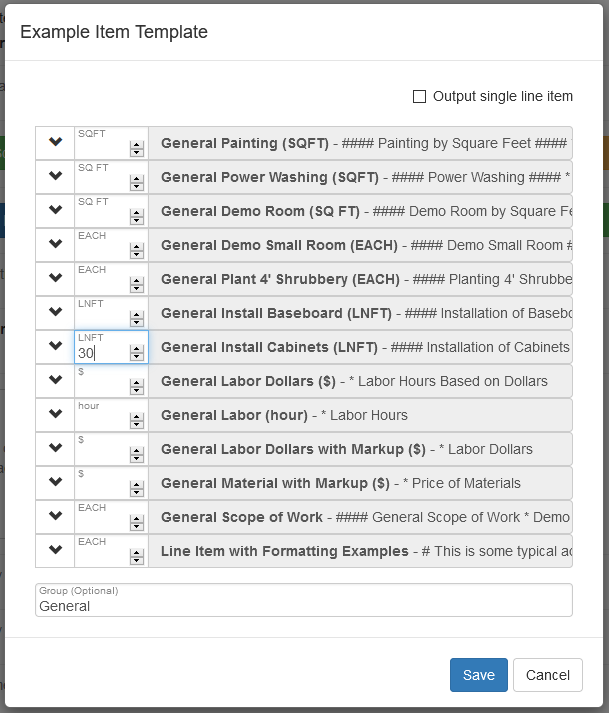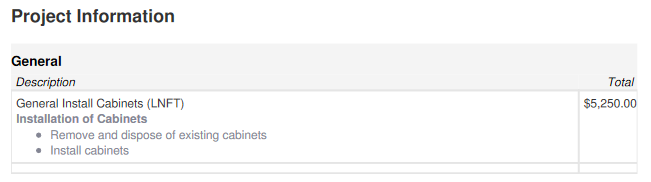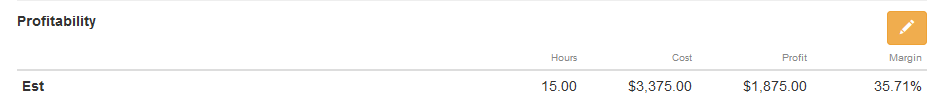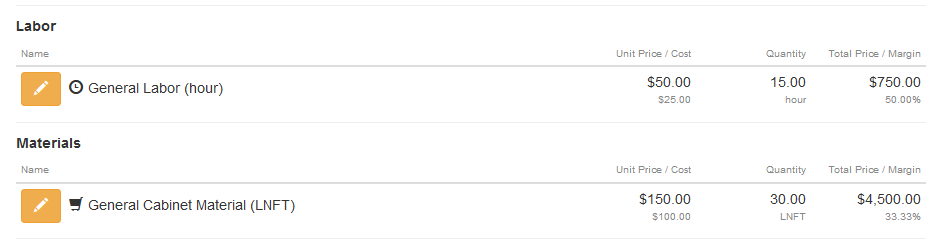# General Install Cabinets (linear-feet) Used in Project

The general install cabinets (linear feet) item assembly is based on a production rate of 2 linear feet per hour. The ideal way to install cabinets may be by each and not linear foot but the concept is the same. The Estimate Rocket smart line items output 3 sets of information when an item is added to a project:

1. Price and detailed work description for your customer to see
2. Estimated profit margin calculation
3. Time and materials calculations for work orders

Click here to see how to modify the general install cabinets (linear feet) for specific use.

In this sample project area is going to have cabinet installation work done

Line Item is Added Directly to the Project• Cabinet measurement = 30 linear feet
• Production rate of installation = 2 linear feet per hour
• Labor Calculation 1 = 30 linear feet / 2 linear feet per hour = 15 hours needed
• Labor Calculation 2 = 15 hours x \$50 per hour = \$750 labor dollars
• Material Calculation 1 = 30 linear feet / 1 linear foot = 30 linear feet of material needed
• Material Calculation 2 = 30 linear feet x \$150 per linear foot of cabinet = \$4500 material dollars
• Total Calculation \$750 labor dollars + \$4500 material dollars = \$5250 total dollarsThe linear footage install cabinets is priced at \$5250 and the customer will see that price on their estimate along with a detailed description of the work to be done.

A Preview of the Estimate shows the customer the price and detailed descriptionEstimated Profit Margin

The general install cabinets also calculates an estimated profit margin for this project as seen in the project detail screen.In this case there are labor and material components involved in the assembly, the price to the customer is \$175.00 per linear foot and the cost is \$112.50 per linear foot. The profit margin for the general install cabinets is 35.71% based on the default item setup.The labor and materials are also calculated for in the work order. The labor and materials section is not displayed on the customer estimate but is displayed in the work order.The labor time and material needed are both calculated based on this assembly.

Click here to see how to modify the general install cabinets (linear feet) for specific use.

Back to the Example Item Template

All Available Item Templates